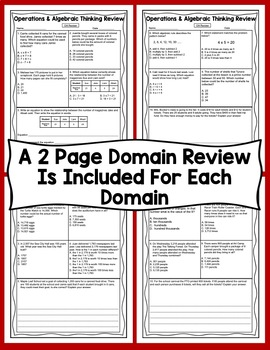# 4th Grade Math Test Prep: All Standards Mega BundleSubject
Resource Type
Format
Zip (43 MB|55 pages)
Standards
\$8.50
Bundle
List Price:
\$13.50
You Save:
\$5.00
\$8.50
Bundle
List Price:
\$13.50
You Save:
\$5.00

### Description

The features of this 4th grade math test prep pack include:

* There is a 1 page review sheet for each 4th Grade Math Common Core Standard

* There is a 2 page comprehensive 4th Grade Common Core Standards test prep review for each domain

* Each review page has 5 questions, 4 questions are multiple choice and 1 is extended response

* The 4th grade test prep review pages compliment daily math instruction and can be used to supplement instruction

* Each page is labeled with the 4th grade math standard for easy organization and data tracking for test prep

★ For more 4th math test prep, check out the following resources:

4th Grade Math Test Prep Mystery Pictures - All Standards Mega Bundle

Math Test Prep - 4th Grade Ultimate Bundle

Math Test Prep (Rock the Test) All Standards Mega Bundle

Math Test Prep (Superhero Theme) All Standards Mega Bundle

4th Grade Common Core Test Prep - All Standards Mega Bundle

4th Grade Math Test Prep Task Cards (Rock the Test) Common Core Mega Bundle

4th Grade Math Test Prep Task Cards Common Core Mega Bundle (Printer Ink Friendly)

4th Grade Math Test Prep Task Cards (Superhero Theme) Common Core Mega Bundle

If you need other 4th grade math materials check out these items:

Math Exit Slips - 4th Grade Common Core All Standards Mega Bundle

4th Grade Common Core Math Assessments - All Standards Bundle

Math Mystery Pictures

Total Pages
55 pages
Included
Teaching Duration
N/A
Report this Resource to TpT
Reported resources will be reviewed by our team. Report this resource to let us know if this resource violates TpT’s content guidelines.

### Standards

to see state-specific standards (only available in the US).
Compare two decimals to hundredths by reasoning about their size. Recognize that comparisons are valid only when the two decimals refer to the same whole. Record the results of comparisons with the symbols >, =, or <, and justify the conclusions, e.g., by using a visual model.
Use decimal notation for fractions with denominators 10 or 100. For example, rewrite 0.62 as 62/100; describe a length as 0.62 meters; locate 0.62 on a number line diagram.
Express a fraction with denominator 10 as an equivalent fraction with denominator 100, and use this technique to add two fractions with respective denominators 10 and 100. For example, express 3/10 as 30/100, and add 3/10 + 4/100 = 34/100.
Recognize a line of symmetry for a two-dimensional figure as a line across the figure such that the figure can be folded along the line into matching parts. Identify line-symmetric figures and draw lines of symmetry.
Classify two-dimensional figures based on the presence or absence of parallel or perpendicular lines, or the presence or absence of angles of a specified size. Recognize right triangles as a category, and identify right triangles.